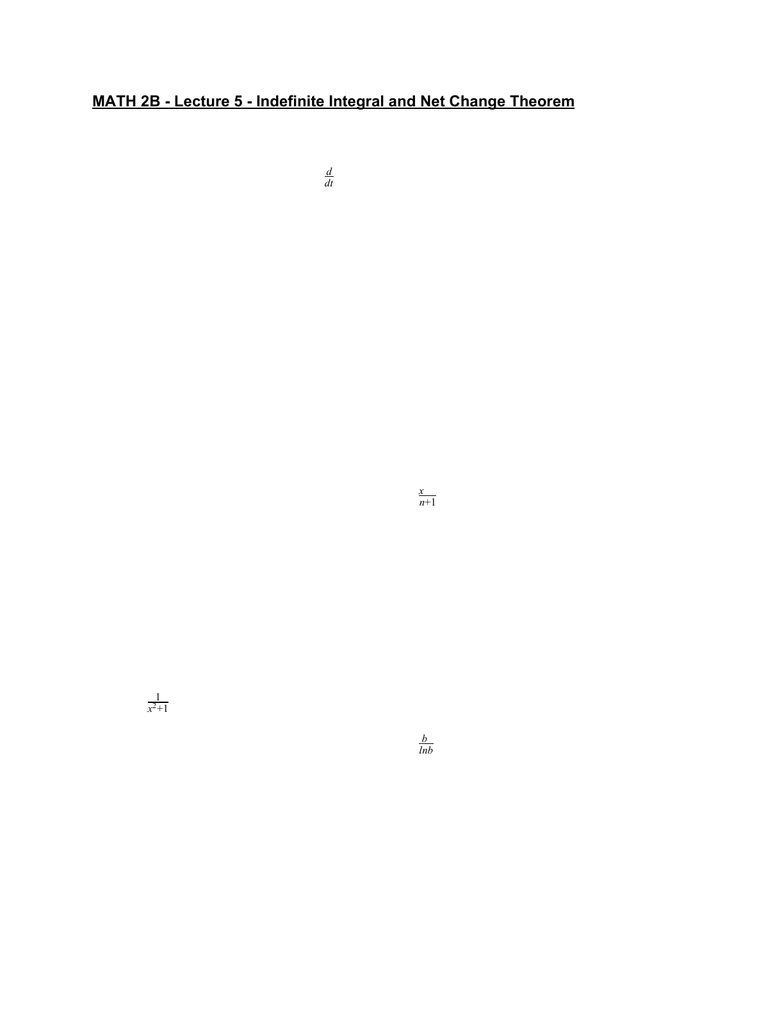Class Notes (1,100,000)
US (460,000)
UC-Irvine (10,000)
MATH (1,000)
MATH 2B (600)
Lecture 5

# MATH 2B Lecture Notes - Lecture 5: AntiderivativePremium

Department
Mathematics
Course Code
MATH 2B
Professor
ERJAEE, G.
Lecture
5

This preview shows half of the first page. to view the full 3 pages of the document.MATH 2B - Lecture 5 - Indefinite Integral and Net Change Theorem
From Fundamental Theorem of Calculus, we know that:
Part I: g’(t) = (x) dx f(t)
d
dt t
a
f=
Part II: (x) dx (x)dx F(b) F(a)
b
a
f=
b
a
F=
which indicates that the derivative and the integral are opposite
operations. We then introduce a new notation for antiderivatives, the
indefinite integral
(x)dx F(x)
f= means F’(x) = fx
Table of indefinite integral for simple functions:
(f(x) (x))dx
+g=(x)dx (x)dx
f+
g
dx
k=xk +c
dx
xn= (n=− )
n+1
xn+1 +c/ 1
dx
ex=ex+c
inx dx
s=osxc+c
ec x dx
s2=anxt +c
ecx tanx dx
s=ec xs +c
dx
1
x+1
2=n|x|l+c
dx
bx=bx
lnb +c
osx dx
c= sinx + c
sc x dx
c2=otxc+c
scx cotx dx
c=scxc+c